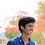In this article, we are going to simulate the Round Robin CPU Scheduling Algorithm using the C Programming Language.
Simulating Round Robin seems more like a pretty complicated program. But the logic is simple once you understand the way each calculation goes. It is easier when you try to understand each line of code and why it is calculated like that. We have seen many codes online for this Round Robin but we were not satisfied with any of those. Especially because those were good codes but didn't contain enough information about the methods used. So, in this article, we are trying to make you understand the logic behind the Round Robin Algorithm and how to simulate it using C programming language. The code might seem complicated. But we have commented literally every single line of code in order to make it extremely easy to understand for everyone.
So, carefully read each line of comment that we gave in the code to understand everything.
The logic is actually pretty simple. The difference comes only when calculating the Waiting time.
So unlike our previous posts in which the other scheduling algorithms were simulated, we are going with a different approach. We are going to use Functions in this to make things easier for you. We have defined functions to calculate the turnaround time and waiting time. Pay closer attention to the function waitingtime(); because that's where most of the important steps are.
For the sake of making it simpler and easier to understand, we are assuming the Arrival time of all processes as 0 in this program.

```//C Program to Simulate Round Robin CPU Scheduling by Nived Kannada
//NOTE: IN THIS, WE ARE ASSUMING THAT ARRIVAL TIME IS 0 FOR ALL PROCESSES.
#include<stdio.h>
//We are defining functions to calculate Turnaroundtime and waiting time.
//Function to find Turnaround time
int turnarroundtime(int p[], int n,
int bt[], int wt[], int tat[]) {
int i;
for (i = 0; i < n ; i++)
tat[i] = bt[i] + wt[i];
return 1;
}
//Function to calculate waiting time
int waitingtime(int p[], int n,
int bt[], int wt[], int quantum)
{
int rem_bt[n];  //rem_bt is remaining burst time for each process(stored as array). It is initially set as equal to bt[].
//Copying bt[] to rem_bt[]
for (int i = 0 ; i < n ; i++)
rem_bt[i] = bt[i];
int t = 0; //Setting initial time as 0
//Traversing processes in Round Robin manner until all of them are not done.
while (1) {
int finished = 1;
for (int i = 0 ; i < n; i++) //Traversing through each process one by one repeatedly.
{
//We have to process only if remaining burst time of a process is greater than zero.
if (rem_bt[i] > 0) //Checking if remaining burst time of current process is greater than zero.
{
finished = 0; // rem_bt[i]>0 means the current process is not finished yet.
if (rem_bt[i] > quantum) {
t = t+quantum;    //Adding time_quantum to current time t.
rem_bt[i] = rem_bt[i] - quantum; //Subtracting time_quantum from remaining burst time.
}
else    // ie. if remaining burst time is less than time_quantum
{
t = t + rem_bt[i];   //adding the remaining burst time with current time t.
wt[i] = t - bt[i];   // Waiting time = current time - burst time of current process.
//in this case, rem_bt[i] is less than the time_quantum.
//ie. process will be finished in this cycle. So we can set rem_bt[i] as 0.
rem_bt[i] = 0;
}
}
}
//If all processes are finished
if (finished == 1)
{  break; }
}
return 1;
}

//Main Function
void main()
{
int n, bt, at,p,wt,tat ,i, total=0, time_quantum; //Here a new variable is introduced --> time_quantum
int x;
float avgwt=0, avgtat=0;

//Reading number of processes from input.
printf("Enter number of processes: ");
scanf("%d",&n);

//Reading Burst times of all processes. (( ASSUMING ARRIVAL TIME IS 0 FOR ALL PROCESSES. ))
printf("Enter the Burst time value of each process: \n");
for(i=0;i<n;i++)
{
p[i]=i;
at[i]=0;
printf("Burst Time of P[%d]: ",i);
scanf("%d",&bt[i]);
}

printf("Enter the Time Quantum: ");
scanf("%d",&time_quantum);

//Calling the functions to calculate waiting time and turnaround time
waitingtime(p, n, bt, wt, time_quantum);
turnarroundtime(p, n, bt, wt, tat);

//Displaying Table
printf("\n\tPID\tAT\tBT\tWT\tTAT\n");
for(i=0;i<n;i++)
{
printf("\t%d\t%d\t%d\t%d\t%d\n",p[i],at[i],bt[i],wt[i],tat[i]);
}

//Calculating Average Waiting time and Average Turnaround Time
for(i=0;i<n;i++)
{
avgwt = avgwt + wt[i];
avgtat = avgtat + tat[i];
}
avgwt = avgwt/n;
avgtat = avgtat/n;

//Displaying Average WT and Average TAT
printf("\nAverage WT = %f\n", avgwt);
printf("\nAverage TAT = %f\n", avgtat);
}

//END OF PROGRAM
```

### Output

1.2.